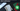# Hackerrank | Solution of Sock Merchant in Golang

April 6th, 2020
|

In this one we will solve question Sock Merchant from hackerrank in golang. Let's dive right into it.

# Problem Statement

The question can be found at this link.

The problem statement states that we are given a list of socks of different colors. We need to find how many pairs of socks exists in the list.

# Solution

The approach here is very simple. We need to find the number of pairs of each color, sum it and return the sum. We can use a map to keep track of all the numbers and their count and in the end calculate the pairs.

For this question since the range of the numbers are very small, we will create a slice of length `101` because the range will only be from `1 to 100` inclusive and slice has zero-based indexing. Next, we will loop over the socks array and for every sock, we will increase its count in the slice i.e. the count at the index represented by sock color in the slice.

After we are done with all the socks, we will loop over the slice and see how many pairs exist in the slice and return it.

``````package main

import "fmt"

func main() {
var n int
fmt.Scan(&n)

socks := make([]int, 101)

for i := 0; i < n; i++ {
var t int
fmt.Scan(&t)

socks[t]++
}

count := 0

for _, v := range socks {
count += v / 2
}

fmt.Println(count)
}``````

Nothing very fancy in the code. We have a socks slice to keep count of all the socks. Next, we find the count of even pairs and return it.

# Summary

So, we solved the sock merchant problem from hackerrank.

I hope you enjoyed solving this question. This is it for this one, complete source code for this post can be found on my Github Repo. Will see you in the next one.